# Machine Learning Basics Building Regression Model In Python

Posted on 2019-03-17, by fubber.

Description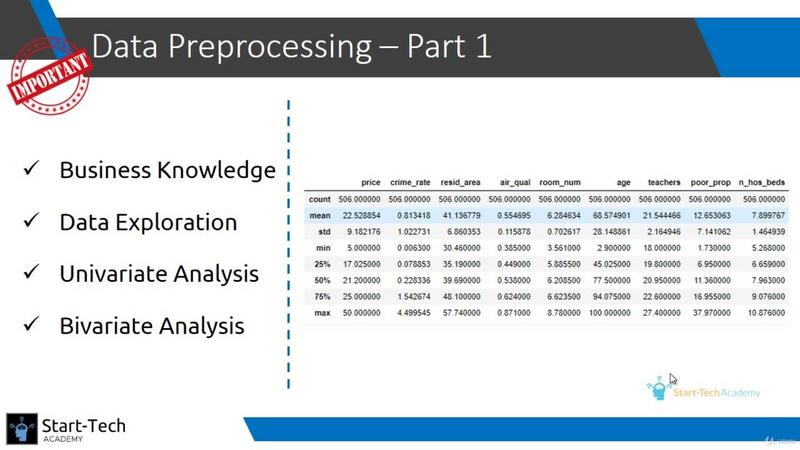Machine Learning Basics Building Regression Model In Python
mp4 | AVC: 1280x720 G_P| Audio:AAC LC 128 Kbps | Total files:158 | mp4, txt, pdf, vtt, csv, html | 2.71 GB
Genre: eLearning | Language: English

General:

What you'll learn

Learn how to solve real life problem using the Linear Regression technique
Preliminary analysis of data using Univariate and Bivariate analysis before running Linear regression
Predict future outcomes basis past data by implementing Simplest Machine Learning algorithm
Understand how to interpret the result of Linear Regression model and translate them into actionable insight
Understanding of basics of statistics and concepts of Machine Learning
Indepth knowledge of data collection and data preprocessing for Machine Learning Linear Regression problem
Learn advanced variations of OLS method of Linear Regression
Course contains a end-to-end DIY project to implement your learnings from the lectures
How to convert business problem into a Machine learning Linear Regression problem
Basic statistics using Numpy library in Python
Data representation using Seaborn library in Python
Linear Regression technique of Machine Learning using Scikit Learn and Statsmodel libraries of Python

Requirements

Students will need to install Python and Anaconda software but we have a separate lecture to help you install the same

Description

The course "Machine Learning Basics: Building Regression Model in Python" teaches you all the steps of creating a Linear Regression model, which is the most popular Machine Learning model, to solve business problems.

Below is a list of popular FAQs of students who want to start their Machine learning journey-

What is Machine Learning?

Machine Learning is a field of computer science which gives the computer the ability to learn without being explicitly programmed. It is a branch of artificial intelligence based on the idea that systems can learn from data, identify patterns and make decisions with minimal human intervention.

What is the Linear regression technique of Machine learning?

Linear Regression is a simple machine learning model for regression problems, i.e., when the target variable is a real value.

Linear regression is a linear model, e.g. a model that assumes a linear relationship between the input variables (x) and the single output variable (y). More specifically, that y can be calculated from a linear combination of the input variables (x).

When there is a single input variable (x), the method is referred to as simple linear regression.

When there are multiple input variables, the method is known as multiple linear regression.

Why learn Linear regression technique of Machine learning?

There are four reasons to learn Linear regression technique of Machine learning:

1. Linear Regression is the most popular machine learning technique

2. Linear Regression has fairly good prediction accuracy

3. Linear Regression is simple to implement and easy to interpret

4. It gives you a firm base to start learning other advanced techniques of Machine Learning

How much time does it take to learn Linear regression technique of machine learning?

Linear Regression is easy but no one can determine the learning time it takes. It totally depends on you. The method we adopted to help you learn Linear regression starts from the basics and takes you to advanced level within hours. You can follow the same, but remember you can learn nothing without practicing it. Practice is the only way to remember whatever you have learnt. Therefore, we have also provided you with another data set to work on as a separate project of Linear regression.

What are the steps I should follow to be able to build a Machine Learning model?

You can divide your learning process into 4 parts:

Statistics and Probability - Implementing Machine learning techniques require basic knowledge of Statistics and probability concepts. Second section of the course covers this part.

Understanding of Machine learning - Fourth section helps you understand the terms and concepts associated with Machine learning and gives you the steps to be followed to build a machine learning model

Programming Experience - A significant part of machine learning is programming. Python and R clearly stand out to be the leaders in the recent days. Third section will help you set up the Python environment and teach you some basic operations. In later sections there is a video on how to implement each concept taught in theory lecture in Python

Understanding of Linear Regression modelling - Having a good knowledge of Linear Regression gives you a solid understanding of how machine learning works. Even though Linear regression is the simplest technique of Machine learning, it is still the most popular one with fairly good prediction ability. Fifth and sixth section cover Linear regression topic end-to-end and with each theory lecture comes a corresponding practical lecture where we actually run each query with you.

Why use Python for data Machine Learning?

Understanding Python is one of the valuable skills needed for a career in Machine Learning.

Though it hasn�t always been, Python is the programming language of choice for data science. Here�s a brief history:

In 2016, it overtook R on Kaggle, the premier platform for data science competitions.

In 2017, it overtook R on KDNuggets�s annual poll of data scientists� most used tools.

In 2018, 66% of data scientists reported using Python daily, making it the number one tool for analytics professionals.

Machine Learning experts expect this trend to continue with increasing development in the Python ecosystem. And while your journey to learn Python programming may be just beginning, it�s nice to know that employment opportunities are abundant (and growing) as well.

What is the difference between Data Mining, Machine Learning, and Deep Learning?

Put simply, machine learning and data mining use the same algorithms and techniques as data mining, except the kinds of predictions vary. While data mining discovers previously unknown patterns and knowledge, machine learning reproduces known patterns and knowledge�and further automatically applies that information to data, decision-making, and actions.

Deep learning, on the other hand, uses advanced computing power and special types of neural networks and applies them to large amounts of data to learn, understand, and identify complicated patterns. Automatic language translation and medical diagnoses are examples of deep learning.

The course is created on the basis of three pillars of learning:

Know (Study)

Do (Practice)

Review (Self feedback)

Know

We have created a set of concise and comprehensive videos to teach you all the Regression related skills you will need in your professional career.

Do

We also provide Exercises to complement the learning from the lecture video. These exercises are carefully designed to further clarify the concepts and help you with implementing the concepts on practical problems faced on-the-job.

Review

Check if you have learnt the concepts by executing your code and analyzing the result set. Ask questions in the discussion board if you face any difficulty.

The Authors of this course have several years of corporate experience and hence have curated the course material keeping in mind the requirement of Regression analysis in today's corporate world.
Who this course is for:

People pursuing a career in data science
Working Professionals beginning their Data journey
Statisticians needing more practical experience
Anyone curious to master Linear Regression from beginner to Advanced in short span of time

Video:

Width: 1280 pixels
Height: 720 pixels
Format: AVC
Codec: AVC
Duration: 00:07:04
Bit rate: 809 Kbps
Frame rate: 30.000 fps
Aspect ratio: 16:9
Bit depth: 8 bits
Color space: YUV
Subtitles: N/A

Audio:

Audio track: 1
Language: N/A
Codec: AAC LC
Channels: 2
Bit rate: 128 Kbps N/A
Compression: Lossy
Sampling rate: 44 Khz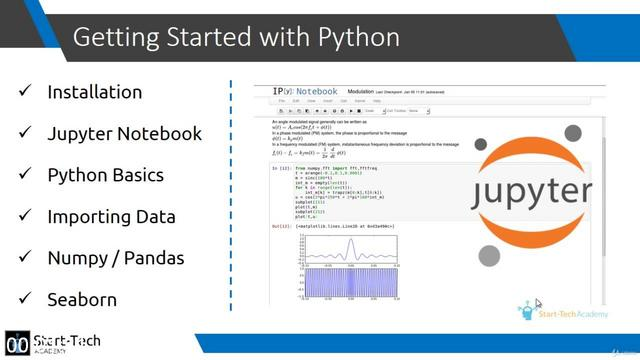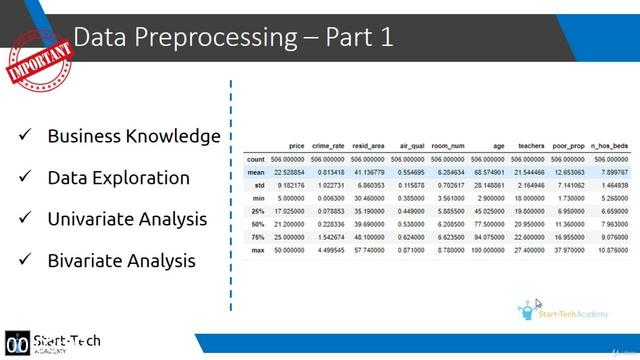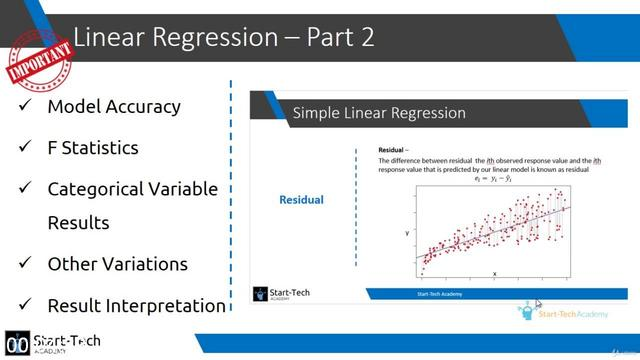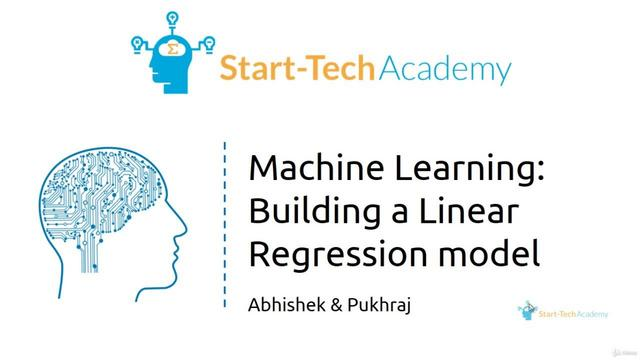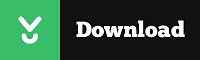`http://nitroflare.com/view/77C1D96DA1CB94B/G_Pk1jpkt41u5t4yj.z01http://nitroflare.com/view/E06BDB512C19BDE/G_Pk1jpkt41u5t4yj.z02http://nitroflare.com/view/95091DA67E05253/G_Pk1jpkt41u5t4yj.z03http://nitroflare.com/view/E919A153B91D62C/G_Pk1jpkt41u5t4yj.z04http://nitroflare.com/view/63D90AF90F50F58/G_Pk1jpkt41u5t4yj.zip`

`https://uploadgig.com/file/download/1708400801c73ce1/G_Pk1jpkt41u5t4yj.z01https://uploadgig.com/file/download/401955Db8772ee41/G_Pk1jpkt41u5t4yj.z02https://uploadgig.com/file/download/20C534d8bdA48d63/G_Pk1jpkt41u5t4yj.z03https://uploadgig.com/file/download/f64400E483ffc4cF/G_Pk1jpkt41u5t4yj.z04https://uploadgig.com/file/download/13a557b7a41A9B2b/G_Pk1jpkt41u5t4yj.zip`

Extract the archives with Winrar 5 or WinZip(zip files) & password

7973 dl's @ 3273 KB/s
8301 dl's @ 2466 KB/s
6034 dl's @ 3573 KB/s

Search More...
Machine Learning Basics Building Regression Model In PythonRelated Books